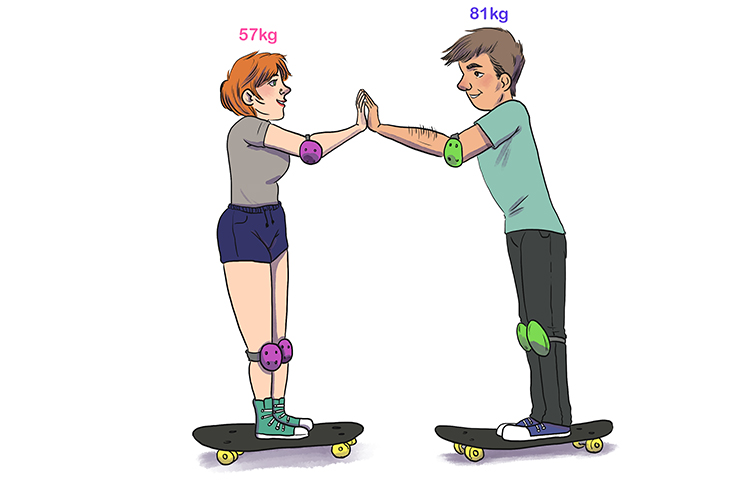# Newton's laws of motion: questions and answers

1. A baseball is thrown at 150m//s^2 and its mass is 0.5kg. What is the force?

F=mxxa

F=0.5kgxx150m//s^2

F=75kgm//s^2

Or

F=75N (Newtons)

2. Two skateboarders stand facing each other.One skateboarder, the woman, weighing 57kg pushes the other skateboarder the man who is 81kg with a force of 85N. What is the acceleration of each skater?

Using Newton's third law, the force on the man must be equal and opposite to the womans, so it must be 85Newtons. We could even say it is minus 85Newtons.

Now using Newton's second law F=ma

Woman

a=F/m

a=(85N)/(57kg)=1.49m//s^2

Man

a=F/m

a=(-85N)/(81kg)=-1.05m//s^2